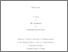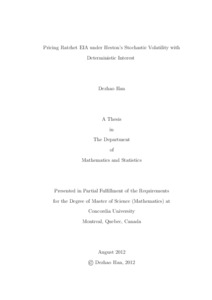Title:

# Pricing Ratchet EIA under Heston’s Stochastic Volatility with Deterministic Interest

Han, Dezhao (2012) Pricing Ratchet EIA under Heston’s Stochastic Volatility with Deterministic Interest. Masters thesis, Concordia University.Preview Text (application/pdf) Han_Dezhao_MSc_F2012.pdf - Accepted Version 1MB

## Abstract

Since its introduction in 1995, equity-indexed annuities (EIAs) received increasing attention from investors. Most of the pricing and hedging for different types of EIAs
have been obtained in the Black-Scholes (BS) framework. In this framework the underlying asset is assumed to follow a geometric Brownian motion. However, the BS model is plagued by its assumption of constant volatility, while stochastic volatility models have become increasingly popular. In this paper we assume that the asset price follows Heston’s stochastic volatility model with deterministic interest, and
introduce two methods to price the ratchet EIA.

The first method is called the joint transition probability density function (JTPDF) method. Given the JTPDF of the asset price and variance, pricing ratchet EIAs boils down to a question of solving multiple integrals. Here, the multiple integral is solved using Quasi Monte Carlo methods and the importance sampling technique. The other method used to evaluate EIAs prices is called the conditional
expectation (CE) approach. Conditioning on the volatility path, we first price the rachet EIA analytically in a BS framework. Then the price in Heston framework can be obtained by simulating the volatility path. Greeks for the ratchet EIA can also be calculated by the JTPDF and CE methods. At the end, we carry out some sensitivity analyses for ratchet EIAs’ prices and Greeks.

Divisions: Concordia University > Faculty of Arts and Science > Mathematics and Statistics Thesis (Masters) Han, Dezhao Concordia University M. Sc. Mathematics 14 September 2012 Gaillardetz, Patrice and José, Garrido 974733 DEZHAO HAN 30 Oct 2012 18:53 18 Jan 2018 17:38
All items in Spectrum are protected by copyright, with all rights reserved. The use of items is governed by Spectrum's terms of access.

Repository Staff Only: item control pageResearch related to the current document (at the CORE website)
Back to top